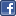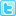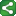Microsoft Excel Resource Center Free Macros, Formulas, Functions, Tutorials, Downloads, Add-Ins & More!
 Monte Carlo Tutorial power4XL Home
 Contents

Modeling the variables to calculate time for commuting to workShareThis

Ultimately we want our model to give output in terms of time - the number of minutes and seconds our commute will take.  Therefore to simplify the model, we'll convert each of the variables to time rather than MPH before adding the variables together.

The first variable in our commuting algorithm is this:  Drive 2 miles on a highway, with 90% probability you will be able to average 65 MPH the whole way, but with a 10% probability that a traffic jam will result in average speed of 20 MPH.  Converting each scenario into time rather than MPH requires simple arithmetic.  Driving 2 miles at 65 MPH requires (60 x 60) x 2 ÷ 65 seconds, or 111 seconds.  At 20 MPH, the time required is (60 x 60) x 2 ÷ 20 seconds, or 360 seconds.  To model the probabilities, we'll use the RAND() function to return a number between 0 and 1.  If the number is less than .9, we'll use the 65 MPH outcome, otherwise we'll use the 20 MPH outcome.  An Excel equation modeling this result is:

=IF(RAND() < .9, 111, 360)

The second variable follows a traffic light through a 120 second cycle where RED lasts 90 seconds and GREEN lasts 30 seconds.  If we hit it on green then there's no delay, but if we hit it on red we wait until it turns green.  This problem is a little tricky because if we make two separate references to RAND() in the equation, each reference will return a different value.  Thus we have to find an equation that uses RAND() only once.  The following equation does the trick:

=MAX(0, (RAND() * 120) - 30)

Let's analyze the equation.  The value "RAND() * 120" inside the inner parentheses simulates the number of seconds remaining in the 120 second cycle after we arrive at the traffic light.  If we arrive during the first 90 seconds of the cycle, we have to wait until the first 90 seconds of the full 120 seconds are elapsed.  This is equal to (TIME REMAINING) - 30.  If we arrive during the last 30 seconds of the cycle, we don't have to wait at all.  In this case, "TIME REMAINING - 30" is a negative number, so MAX(0, TIME REMAINING) returns 0 wait time.

Recapping the third leg of the commute: "Travel 2 more miles on a surface street.  70% of the time you travel at 30 MPH.  10% of the time you average 20 MPH, 10% of the time you average 40 MPH, and 10% of the time there's a traffic jam that takes you 30 minutes to travel these two miles."

This third variable is similar to the first, but contains four scenarios instead of two.  Therefore we will use  "nested if" statements to handle the second, third and fourth scenarios.  Converting the four scenarios into seconds gives us the following:

• (60 x 60) x 2 ÷ 30 = 240

• (60 x 60) x 2 ÷ 20 = 360

• (60 x 60) x 2 ÷ 40 = 180

• 30 x 60 = 1800

Now we construct the Excel equation to give us each of the above outcomes according to their probability.  Again we have the limitation of using only one instance of the RAND() function.  One way we can achieve this is by using the CHOOSE() function as follows:

=CHOOSE(INT(RAND()*10) + 1, 240, 240, 240, 240, 240, 240, 240, 360, 180, 1800)

Another way to achieve the same result is by using a lookup table.  We can create the lookup table as follows:

The Excel equation for the third variable using the lookup table approach is as follows:

=VLOOKUP(RAND(), \$C\$8:\$D\$11, 2)

In our construction of the model on the next page we will use the CHOOSE() function approach.

Copyright notice:  This site and all content, including computer code and spreadsheet examples, are copyright 2006 by Fritz Dooley.  License is granted for individual users to download examples and to copy code directly into user's spreadsheets and Visual Basic for Applications files.  Users may not redistribute code in any way.  Providing hyperlinks to this web site is encouraged, but posting code and examples on other web sites is expressly forbidden.  "Microsoft" is a registered trademark of Microsoft Corporation.   Neither this web site nor Fritz Dooley is affiliated with Microsoft Corporation.